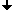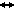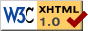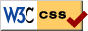﻿ Seminar: Dynamics of traveling waves, Winter 2014/15
Nonlinear Dynamics at the Free University Berlin
Winter 2014/15

# Seminar: Dynamics of traveling waves

PD Dr. Pavel Gurevich,

## Schedule, Winter 2014/15

Seminar:
Friday 10.00-12.00, SR 130/ Arnimallee 3

## Description

Traveling waves arise in many fields of natural sciences, including biology, chemistry, and physics. We will learn mathematical tools that allow one to discribe the dynamics of traveling waves in reaction-diffusion systems. Besides classical systems, we will be interested in those containing time delay and spatially nonlocal interactions.

The language of the seminar is supposed to be English (with the help of German if needed).

Knowledge of ordinary differential equations and/or dynamical systems is necessary. Basic understanding of partial differential equations will be helpful.

## Topics

KPP and Fisher equations
1. Existence of traveling fronts for bistable Fisher (Nagumo) equation. Phase-plane method.
1. A. I. Volpert, Vit. A. Volpert, Vl. A. Volpert. Traveling wave solutions of parabolic systems, Translations of Mathematical Monographs, Vol. 140 (1994),448 pp., American Mathematical Society, Providence, RI (Chapter 1)
2. D Henry. Geometric Theory of Semilinear Parabolic Equations Lecture Notes in Math., Vol. 240 Springer-Verlag, Berlin (1981). (Section 5.4)
2. Existence of traveling fronts for monostable Kolmogorov-Piskunov-Petrovskii (KPP) equation. Phase plane method.
1. A. I. Volpert, Vit. A. Volpert, Vl. A. Volpert. Traveling wave solutions of parabolic systems, Translations of Mathematical Monographs, Vol. 140 (1994), 448 pp., American Mathematical Society, Providence, RI (Chapter 1)
2. A. N. Kolmogorov, I. G. Petrovskii and N. S. Piskunov, A study of the diffusion equation with increase in the quantity of matter, and its application to a biological problem, Bull. Moscow Univ. Math. ser. A 1, 1-25 (1937).
3. Stability of traveling fronts
1. D Henry. Geometric Theory of Semilinear Parabolic Equations Lecture Notes in Math., Vol. 240 Springer-Verlag, Berlin (1981). (Chapter 5, Section 5.4)
2. A. I. Volpert, Vit. A. Volpert, Vl. A. Volpert. Traveling wave solutions of parabolic systems, Translations of Mathematical Monographs, Vol. 140 (1994), 448 pp., American Mathematical Society, Providence, RI (Chapter 4,5)
4. Traveling fronts for KPP and Fisher equations. Leray-Shauder method
1. A. I. Volpert, Vit. A. Volpert, Vl. A. Volpert. Traveling wave solutions of parabolic systems, Translations of Mathematical Monographs, Vol. 140 (1994),448 pp., American Mathematical Society, Providence, RI (Chapter 2,3)
Systems with time delay
5. Traveling fronts and their stability
1. K. Schaaf. Asymptotic behavior and traveling wave solutions for parabolic functional differential equations. Trans.-Am. Math. Soc. 302 (1987), 587-615.
2. D Henry. Geometric Theory of Semilinear Parabolic Equations Lecture Notes in Math., Vol. 240 Springer-Verlag, Berlin (1981). (Chapter 5, Section 5.4)
Systems with spatially nonlocal interactions
6. Traveling fronts and their stability
1. P. W. Bates, P. C. Fife, X. Ren, X. Wang: Traveling waves in a convolution model for phase transitions, Arch. Rational Mech. Anal. 138, 105-136 (1997).
7. Propagation speed of traveling fronts
1. J. Coville and L. Dupaigne, Propagation speed of traveling fronts in non local reaction-diffusion equations, Nonlinear Analysis 60 (2005), pp. 797-819.
2. P. W. Bates, P. C. Fife, X. Ren, X. Wang: Traveling waves in a convolution model for phase transitions, Arch. Rational Mech. Anal. 138, 105-136 (1997).
8. Absence of comparison principle and nonmonotone fronts. Bistable case.
1. M. Alfaro, J. Coville, G. Raoul. Bistable traveling waves for nonlocal reaction diffusion equations arXiv:1303.3554 [math.AP] (2013).
FitzHugh-Nagumo systems
9. Existence of pulses
1. G.A. Carpenter, A geometric approach to singular perturbation problems with applications to nerve impulse equations Journal of Differential Equations 23: 335 (1977)
2. Hastings, S. P. On the existence of homoclinic and periodic orbits for the Fitzhugh-Nagumo equations. Quart. J. Math. Oxford Ser. (2) 27 (1976), no. 105, 123-134.
3. E. M Izhikevich. Dynamical Systems in Neuroscience: The Geometry of Excitability and Bursting. The MIT Press, Cambridge, MA (2007).
4. J. Nagumo, S. Arimoto, and S. Yoshizawa. An active pulse transmission line simulating nerve axon. Proc IRE. 50 (1962), 2061-2070.
5. B. Deng, The existence of infinitely many traveling front and back waves in the FitzHugh-Nagumo equations, SIAM J. Math. Anal. 22 (1991), 1631-1650.
6. W. Gao, J. Wang. Existence of wavefronts and impulses to FitzHugh-Nagumo equations. Nonlinear Anal. 57 (2004), no. 5-6, 667-676.
10. Stability of pulses
1. Ch. K. R. T. Jones Stability of the traveling wave solution of the Fitzhugh-Nagumo system. Trans. Amer. Math. Soc. 286 (1984), 431-469.
11. Pulses in nonlocal Fitzhugh-Nagumo systems
1. G. Faye, A. Scheel. Existence of pulses in excitable media with nonlocal coupling, arXiv:1311.6508 [math.AP] (2013).
12. Spiral waves
1. A. Scheel. Bifurcation to spiral waves in reaction-diffusion systems SIAM J. Math. Anal. 29 (1998), 1399-1418Last change: Sep. 17, 2014
 This page strictly conforms to the XHTML1.0 standard and uses style sheets.